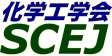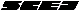## \$B9V1i%W%m%0%i%`!J2q>l!&F|DxJL!K(B

### V\$B2q>l(B \$BBh(B2\$BF|(B

\$B:G=*99?7F|;~!'(B2012-08-20 15:02:17
\$B9V1i(B
\$B;~9o(B
\$B9V1i(B
\$BHV9f(B
\$B9V1iBjL\!?H/I=\$B%-!<%o!<%I(B\$BJ,N`(B
\$BHV9f(B
\$B\$BHV9f(B
\$B%7%s%]%8%&%`(B \$B!cJ,N%Kl!&Kl%W%m%;%93+H/\$N?7E83+!d(B
(13:00\$B!A(B14:00)\$B!!(B(\$B:BD9(B \$BCfB<(B \$B0lJf(B)
13:00\$B!A(B 13:20V213MPC\$B%]%j%^!<=hM}%;%i%_%C%/Kl\$NBQ%U%!%&%j%s%0@-I>2A(B
(\$B?@F`@n9)Bg1~MQ%P%\$%*2J(B) \$B!{(B(\$B@5(B)\$B;TB<(B \$B=E=S(B \$B!&(B \$BBgLn(B \$B@5><(B \$B!&(B \$B@P@n(B \$BBnLi(B \$B!&(B (\$BElBg1!9)(B) \$B@P86(B \$B0lI'(B
fouling
2-Methacryloyloxyethyl phosphorylcholine polymer
ceramic membrane
S-21643
13:20\$B!A(B 13:40V214\$B%W%i%:%^%0%i%U%H=E9gK!\$rMQ\$\$\$F:n@=\$7\$?(Bpoly(2-methoxyethylacrylate)\$B8GDjKl\$NDc%U%!%&%j%s%0@-(B
(\$B9)3X1!Bg9)(B) \$B!{(B(\$B@5(B)\$B@V>>(B \$B7{ \$B!&(B \$B4Z(B \$BK'(B \$B!&(B \$B8E9>(B \$BN4L@(B \$B!&(B (\$B@5(B)\$BCfHx(B \$B??0l(B
fouling
plasma graft polymerization
2-methoxyethylacrylate
S-21128
13:40\$B!A(B 14:00V215\$B%+%k%\%-%7%a%A%k%Y%?%\$%s7O%]%j%^!<\$N?eOB>uBV\$HDc%U%!%&%j%s%0@-(B
(\$B9)3X1!Bg9)(B) \$B!{(B(\$B@5(B)\$B@V>>(B \$B7{ \$B!&(B \$B1|;3(B \$B\$_\$f\$-(B \$B!&(B (\$B@5(B)\$BCfHx(B \$B??0l(B
zwitterionic
fouling
hydration
S-21129
(14:00\$B!A(B15:20)\$B!!(B(\$B:BD9(B \$B;TB<(B \$B=E=S(B)
14:00\$B!A(B 14:20V216Improvement of performance of anion exchange membrane for electrodialysis by LbL
(Kobe U.) \$B!{(B(\$B3X(B)Sri Mulyati \$B!&(B Takagi Ryosuke \$B!&(B (\$B@5(B)Ohmukai Yoshikage \$B!&(B (\$B@5(B)Matsuyama Hideto
antifouling potential
monovalent selectivity
electrodialysis
S-21323
14:20\$B!A(B 14:40V217DMFC\$B\$X\$NMxMQ\$K8~\$1\$?%]%j%9%A%l%s%9%k%[%s;@(B/\$B%0%j%3!<%k%-%H%5%sJ#9gKl\$N3+H/(B
(\$BElM}Bg1!Am2=(B) \$B!{(B(\$B3X(B)\$BKY>>(B \$BD>9d(B \$B!&(B (\$BElM}Bg9)(B) (\$B@5(B)\$B9b66(B \$BCR51(B \$B!&(B (\$B@5(B)\$B>.NS(B \$BBgM4(B \$B!&(B (\$B@5(B)\$B>1Ln(B \$B8|(B \$B!&(B (\$B@5(B)\$BBgC](B \$B>!?M(B
direct methanol fuel cell
polyelectrolyte membrane
chitosan
S-21452
(\$B2#9qBg9)(B) \$B!{(B(\$B@5(B)\$BCfB<(B \$B0lJf(B \$B!&(B (\$B@5(B)\$B>>K\(B \$B44<#(B
fouling
zeta potential
microfiltration
S-21858
15:00\$B!A(B 15:20V219\$BN3;R7B\$N0[\$J\$k:.9g%J%NN3;RJ,;61U\$N%@%\$%J%_%C%/8B30_I2aFC@-(B
(\$BL>Bg9)(B) \$B!{(B(\$B@5(B)\$B8~0f(B \$B9/?M(B \$B!&(B \$B:4F#(B \$B>=(B \$B!&(B \$Bjj(B \$B3\$1m(B
nanoparticle
dynamic filtration
binary mixture
S-21882

\$B9V1i%W%m%0%i%`(B
\$B2=3X9)3X2q(B \$BBh(B44\$B2s=)5(Bg2q(B(C) 2012 \$B8x1W
Most recent update: 2012-08-20 15:02:17
For more information contact \$B2=3X9)3X2qElKL;YIt(B \$BBh(B44\$B2s=)5(Bg2q(B \$BLd\$\$9g\$;78(B
E-mail: inquiry-44fwww3.scej.org
This page was generated byeasp 2.28; proghtml 2.28c (C)1999-2011 kawase